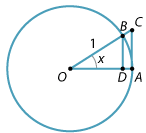## Content

### An important limit

In order to apply calculus to the trigonometric functions, we will need to evaluate the fundamental limit

$\lim_{x\to 0} \dfrac{\sin x}{x},$

which arises when we apply the definition of the derivative of $$f(x) = \sin x$$.

It must be stressed that, from here on in this module, we are measuring $$x$$ in radians.

You can see that attempting to substitute $$x=0$$ into $$\dfrac{\sin x}{x}$$ is fruitless, since we obtain the indeterminate form 'zero over zero'. On the other hand, if we try substituting say $$x=0.1$$ (in radians), then the calculator gives $$0.998\dots$$, and if we try $$x=0.01$$, we get $$0.99998\dots$$.

We guess that, as $$x$$ approaches 0, the value of $$\dfrac{\sin x}{x}$$ approaches 1. We will now give a nice geometric proof of this fact.

Consider a sector $$OAB$$ of the unit circle containing an acute angle $$x$$, as shown in the following diagram. Drop the perpendicular $$BD$$ to $$OA$$ and raise a perpendicular at $$A$$ to meet $$OB$$ produced at $$C$$. Since $$OA = OB = 1$$, we have $$BD = \sin x$$ and $$AC = \tan x$$.Detailed description

The area of the sector $$OAB$$ is $$\dfrac{1}{2} x$$. The sector $$OAB$$ clearly contains the triangle $$OAB$$ and is contained inside the triangle $$OAC$$. Hence, comparing their areas, we have

\begin{align*} \text{Area } \triangle OAB \leq \dfrac{1}{2} x \leq \text{Area } \triangle OAC \quad &\implies \quad \dfrac{1}{2}OA \cdot BD \leq \dfrac{1}{2} x \leq \dfrac{1}{2}OA \cdot AC \\ &\implies \quad \dfrac{1}{2}\sin x \leq \dfrac{1}{2} x \leq \dfrac{1}{2}\tan x \\ &\implies \quad \sin x \leq x \leq \tan x. \end{align*}

Now, since $$\tan x = \dfrac{\sin x}{\cos x}$$ and since $$\sin x > 0$$ for $$0 < x < \dfrac{\pi}{2}$$, we can divide both inequalities by $$\sin x$$ to obtain

$1 \leq \dfrac{x}{\sin x} \leq \dfrac{1}{\cos x}.$

Since $$\cos x$$ approaches 1 as $$x$$ approaches 0, we see that

$\dfrac{x}{\sin x} \to 1 \quad\text{as}\quad x\to 0.$

Taking the reciprocal, it follows that

$\dfrac{\sin x}{x} \to 1 \quad\text{as}\quad x\to 0.$ (Here we have used the pinching theorem and the algebra of limits, as discussed in the module Limits and continuity.)

Thus we can write

$\lim_{x\to 0} \dfrac{\sin x}{x} = 1.$

Note that this means that, if the angle $$x$$ is small, then $$\sin x \approx x$$. This fact is often used by physicists when analysing such things as a simple pendulum with small angle.

Exercise 1

Using the limit above, prove that

$\lim_{x\to 0} \dfrac{\tan x}{x} = 1.$

Other related limits can be found by manipulating this basic limit.

#### Example

Find

$\lim_{x\to 0} \dfrac{\sin 2x}{3x}.$

#### Solution

We can write

$\dfrac{\sin 2x}{3x} = \dfrac{\sin 2x}{2x} \times \dfrac{2}{3}.$

If we let $$u=2x$$, then as $$x\to 0$$, we have $$u\to 0$$. Hence

$\lim_{x\to 0} \dfrac{\sin 2x}{3x} = \dfrac{2}{3} \lim_{u\to 0} \dfrac{\sin u}{u} = \dfrac{2}{3}.$

Exercise 2

Find

$\lim_{x\to 0} \dfrac{\sin 3x + \sin 7x}{5x}.$

Exercise 3

1. Show that $$\lim\limits_{x\to 0} \dfrac{1-\cos x}{x^2} = \dfrac{1}{2}$$.
2. Deduce that $$\cos x \approx 1-\dfrac{1}{2}x^2$$, for small $$x$$.

Next page - Content - Differentiating trigonometric functions